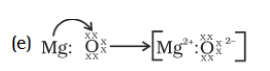# An element is placed in 2nd Group and 3rd Period of the Periodic Table, burns in presence of oxygen to form a basic oxide. (a) Identify the element (b) Write the electronic configuration (c) Write the balanced equation when it burns in the presence of air (d) Write a balanced equation when this oxide is dissolved in water (e) Draw the electron dot structure for the formation of this oxide

(a) Element is Magnesium

(b) Electronic Configuration of magnesium is written as

The number of electrons for the Mg atom are 12 electrons. The electron configuration of magnesium is,

Mg (Z= 12) = 1s2 2s2 2p6 3s2

• The first two electrons are placed in the 1s orbital. The 1s orbital can accommodate two electrons.
• The next 2 electrons for magnesium go in the 2s orbital.
• The next six electrons will go in the 2p orbital. The p orbital can hold up to six electrons.
• We’ll put six in the 2p orbital and then put the remaining two electrons in the 3s.

(c) The balanced equation when magnesium burns in the presence of air

When magnesium is burnt in the air it forms magnesium oxide(MgO)

2Mg + O2 → 2MgO

(d) The balanced equation when magnesium oxide is dissolved in water

MgO + H2O → Mg(OH)2

(e) The electron dot structure for the formation of magnesium oxide(0)(0)